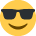# Irr

Hi all,

I have noticed from most practice papers that an explaination of IRR is always in there. Can anyone help in plain english how this works?

Thanks
Vicky

• To Understand IRR we need to understand the NPV.

NPV is a value of the FUTURE income worth today at a given (or expected) return rate on investment.

Therefore if I project to have a balance of £1100 in my current account by the end of year and my expectation of return on my investment is 10%, how much should I invest at the beginning of the year?

Of course the answer is £1000.

If then I am offered an alternative investment and I am promised a return £1100 but I only have to invest 950? Then I am getting a discount of £50 at today’s price.

Now let us understand IRR

If I am getting £1100 in 12 months time and I only have to invest £950 today what is the interest I am receiving?

We know that the answer is that at IRR discount is Zero. If we apply interest rate of 15.8% on our investment, the interest earned on £950 is £150 a making the compounded amount of £1100

At 15.8% interest rate and investment of £950 will result in zero discount at today’s price.

Therefore the IRR rate is 15.8%
• Hi All,

I'm glad i'm not the only one struggling with IRR.

I understand NPV (i think!) This is what future income from your investment would be worth today calculated at a given rate of return.

But i am still confused about IRR. I understand that it is when the return is brought back to zero. So does this mean that the IRR percentage is the amount that interest rates could rise to before a loss would be made on the investment. Or does it mean that that is the percentage return you would expect?

Or am i just getting confused even more!

I know all we are really expected is to be able to say what the IRR is, but i don't want to just memorise something that i don't understand!

Ampsie
:001_unsure:
• Ampsie wrote: »
A) So does this mean that the IRR percentage is the amount that interest rates could rise to before a loss would be made on the investment.Or does it mean that that is the percentage return you would expect?

I agree with A not with B. The first one is the definition you find in the text books. Remember though, for the purposes of the calculations we assume that the interest rate on any loans are fixed at the start of the project - they are not allowed to change over time.

We don't really know the expected return because we don't know the actual cost of capital. In practice a company may set a target IRR of 15% say, expecting a cost of capital of 10% and a profit margin of 5%.

Hope this helps! :001_smile:
• Aha!

So the IRR shows the rate of return on the capital when income equals expenditure.

I think it is slowly beginning to sink in!!

Ampsie
• Ampsie wrote: »
Aha!

So the IRR shows the rate of return on the capital when income equals expenditure.

I think it is slowly beginning to sink in!!

Ampsie
Net Present value is the value of the future income of the investment? Incase if the exam question ask what is NVP? please PM me.

IRR is the another method of the apprisal investment capital of cost. It is used for discount rate which caused NVP to be zero. it is the maximum cost of the investment for the project so we wil lnot need to reduce the shareholder's value. Is it correct as i typed this without cheating or looking at the answer.